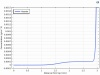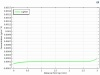# 用于毒理学分析的实用沉降仿真 App

2022年 1月 25日### 重力作用下的试管沉降方程\frac
{\partial c}{\partial t} – \frac{\partial}{\partial x} \left ( A \frac{\partial c} {\partial x} + Bgc \right) =0, \ (x,t) \in [0,x_2] \times [0,T]

A \frac{\partial c}{\partial x}+ Bgc=0, \ \ x = 0, \ x_2

c = c_0, \hspace{0.5cm}\ \ t=0,

Mason-Weaver 方程是一个容易理解和求解的方程，除了极快的沉降情况。让我们试着解释一下它的物理意义。它由两个方程组成：

1. 扩散（第二项）
2. 重力对流（第三项）### 粒度分布颗粒沉降仿真 App

#### 步骤1#### 步骤2#### 步骤3### 附加说明

A = \frac{k_B T} {6 \pi \eta r},

B=\frac{2g(\rho_e-\rho_s)r^2} {9\eta},

### 参考文献

1. G.M. DeLoid et al., “Advanced computational modeling for in vitro nanomaterial dosimetry”, Particle and fibre toxicology, vol. 12, no. 1, pp. 1–20, 2015.
2. K. Christmann, Introduction to Surface Physical Chemistry, Springer Science & Business Media, vol. 1., 2013.
3. K.H. Ramteke et al., “Mathematical models of drug dissolution: a review”, Sch. Acad. J. Pharm, vol. 3, no. 5, pp. 388–396, 2014.
4. A. Dokoumetzidis and P. Macheras, “A century of dissolution research: from Noyes and Whitney to the biopharmaceutics classification system”, International Journal of Pharmaceutics 321.1-2 (2006): 1–11.

Oracle and Java are registered trademarks of Oracle and/or its affiliates.

#### 评论 (2)

##### 留言##### 中泉 高
2023-04-12##### Yosuke Mizuyama
2023-04-12 COMSOL 员工